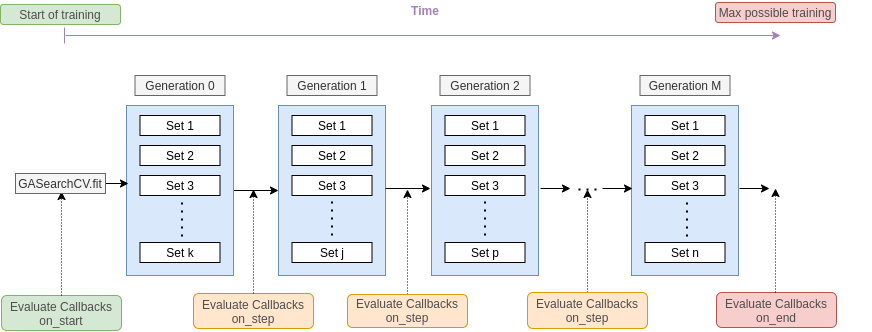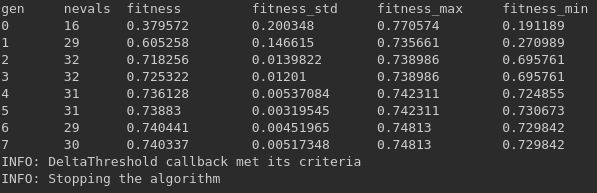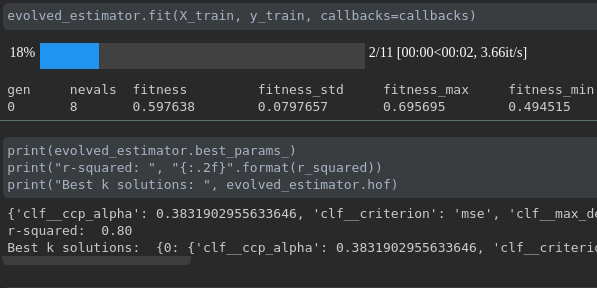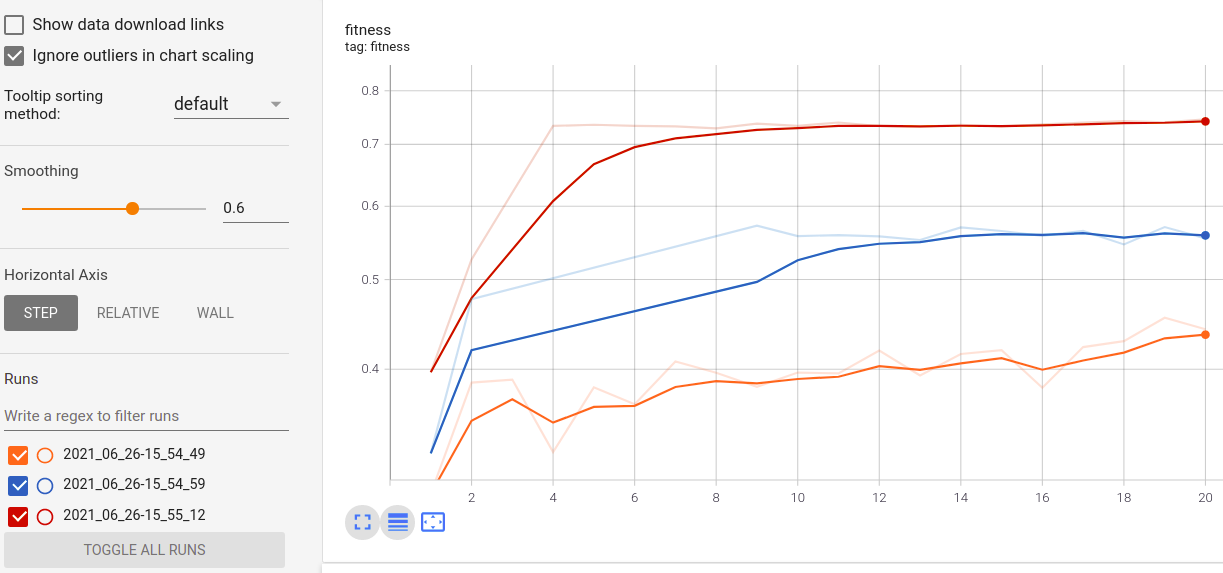# Using Callbacks¶

## Introduction¶

Callbacks can be defined to take actions or decisions over the optimization process while it is still running. Common callbacks include different rules to stop the algorithm or log artifacts. The callbacks are passed to the `.fit` method of the `GASearchCV` class.

The callbacks are evaluated at the start of the training using the on_start method, at the end of each generation fit using on_step method and at the end of the training using on_end, so it looks like this:When a stopping callback condition is met, we should see a message like this and the model must stop. It will keep all the information until that training point.Now let’s see how to use them, we’ll take the data set and model used in How to Use Sklearn-genetic-opt. The available callbacks are:

• ProgressBar

• ConsecutiveStopping

• DeltaThreshold

• TimerStopping

• ThresholdStopping

• TensorBoard

• LogbookSaver

## ProgressBar¶

This callback display a tqdm bar with your training process, the length of the bar is the max number of generations (population_size + 1) that the algorithm would run, each step is a generation.

You can pass any `tqdm.auto.tqdm` valid arguments as kwargs or leave it as default. To use this bar set:

```from sklearn_genetic.callbacks import ProgressBar
callback = ProgressBar()
```

Now we just have to pass it to the estimator during the fitting

```# Already defined GASearchCV instance
evolved_estimator.fit(X, y, callbacks=callback)
```

During the training it will be displayed like this:## ConsecutiveStopping¶

This callback stops the optimization if the current metric value is no greater than at least one metric from the last N generations.

It requires us to define the number of generations to compare against the current generation and the name of the metric we want to track.

For example, if we want to stop the optimization after 5 iterations where the current iteration (sixth) fitness value is worst than all the previous ones (5), we define it like this:

```from sklearn_genetic.callbacks import ConsecutiveStopping
callback = ConsecutiveStopping(generations=5, metric='fitness')
```

Now we just have to pass it to the estimator during the fitting

```# Already defined GASearchCV instance
evolved_estimator.fit(X, y, callbacks=callback)
```

## DeltaThreshold¶

Stops the optimization if the absolute difference between the maximum and minimum value from the last N generations is less or equals to a threshold.

The threshold gets evaluated after the number of generations specified is reached; the default number is 2 (the current and previous one).

It just requires the threshold, the metric name and the generations, for example using the ‘fitness_min’ value and comparing the last 5 generations:

```from sklearn_genetic.callbacks import DeltaThreshold
callback = DeltaThreshold(threshold=0.001, generations=5, metric='fitness_min')

evolved_estimator.fit(X, y, callbacks=callback)
```

## TimerStopping¶

This callback stops the optimization if the difference in seconds between the starting time of the first set of hyperparameters fit, and the current generation time is greater than a time threshold.

Remember that this is checked after each generation fit, so if the first (or any) generation fit takes longer than the threshold, it won’t stop the fitting process until is done with the current generation population.

It requires the total_seconds parameters, for example stopping if the time is greater than one minute:

```from sklearn_genetic.callbacks import TimerStopping
callback = TimerStopping(total_seconds=60)

evolved_estimator.fit(X, y, callbacks=callback)
```

## ThresholdStopping¶

It stops the optimization if the current metric is greater or equals to the defined threshold.

For example, if we want to stop the optimization if the ‘fitness_max’ is above 0.98:

```from sklearn_genetic.callbacks import ThresholdStopping
callback = ThresholdStopping(threshold=0.98, metric='fitness_max')

evolved_estimator.fit(X, y, callbacks=callback)
```

## TensorBoard¶

It saves at each iteration the fitness metrics into a log folder that can be read by Tensorboard.

To use this callback you must install tensorflow first, this is not installed within this package due it’s usually a sensitive and heavy dependency:

```pip install tensorflow
```

It only requires defining the folder where you want to log your run, and optionally, a run_id, so your consecutive runs don’t mix up. If the run_id is not provided, it will create a subfolder with the current date-time of your run.

```from sklearn_genetic.callbacks import TensorBoard
callback = TensorBoard(log_dir="./logs")

evolved_estimator.fit(X, y, callbacks=callback)
```

While the model is being trained you can see in real-time the metrics in Tensorboard. If you have run more than one GASearchCV model and use the TensordBoard callback using the same log_dir but different run_id, you can compare the metrics of each run, it looks like this for the fitness in three different runs:## LogbookSaver¶

It saves at each iteration the Logbook object with all the parameters and the cv-score achieved by those parameters. It uses joblib.dump to save the file.

```from sklearn_genetic.callbacks import LogbookSaver
callback = LogbookSaver(checkpoint_path="./logbook.pkl")

evolved_estimator.fit(X, y, callbacks=callback)
```

Then the object can be restored:

```from joblib import load

print(logbook)
```

## Define Multiple Callbacks¶

You can also specify more than one callback at the same time. The way to define it is by passing a list of callbacks in the `.fit` method.

Then the estimator is going to check all the conditions in every iteration, if at least one of the stopping callbacks conditions is met, the callback will stop the process:

```from sklearn_genetic.callbacks import ThresholdStopping, DeltaThreshold
threshold_callback = ThresholdStopping(threshold=0.98, metric='fitness_max')
delta_callback = DeltaThreshold(threshold=0.001, metric='fitness')

callbacks = [threshold_callback, delta_callback]

evolved_estimator.fit(X, y, callbacks=callbacks)
```

## Full Example¶

This example uses a ThresholdStopping and DeltaStopping callback It will stop if the accuracy of the generation is above 0.98 or if the difference between the current generation accuracy and the last generation accuracy is not bigger than 0.001:

```from sklearn_genetic import GASearchCV
from sklearn_genetic.space import Categorical, Integer, Continuous
from sklearn.model_selection import train_test_split, StratifiedKFold
from sklearn.tree import DecisionTreeClassifier
from sklearn.metrics import accuracy_score
from sklearn_genetic.callbacks import ThresholdStopping, DeltaThreshold

label_names = data['target_names']
y = data['target']
X = data['data']

X_train, X_test, y_train, y_test = train_test_split(X, y, test_size=0.33, random_state=42)

clf = DecisionTreeClassifier()

params_grid = {'min_weight_fraction_leaf': Continuous(0, 0.5),
'criterion': Categorical(['gini', 'entropy']),
'max_depth': Integer(2, 20), 'max_leaf_nodes': Integer(2, 30)}

cv = StratifiedKFold(n_splits=3, shuffle=True)

threshold_callback = ThresholdStopping(threshold=0.98, metric='fitness_max')
delta_callback = DeltaThreshold(threshold=0.001, metric='fitness')

callbacks = [threshold_callback, delta_callback]

evolved_estimator = GASearchCV(clf,
cv=cv,
scoring='accuracy',
population_size=16,
generations=30,
tournament_size=3,
elitism=True,
crossover_probability=0.9,
mutation_probability=0.05,
param_grid=params_grid,
algorithm='eaMuPlusLambda',
n_jobs=-1,
verbose=True)

evolved_estimator.fit(X_train, y_train, callbacks=callbacks)
y_predict_ga = evolved_estimator.predict(X_test)
accuracy = accuracy_score(y_test, y_predict_ga)

print(evolved_estimator.best_params_)
print("accuracy score: ", "{:.2f}".format(accuracy))
```